# Electronics and Communication Engineering - Analog Electronics

6.

An RC coupled amplifier has an open loop gain of 200 and a lower cutoff frequency of 50 Hz. If negative feedback with β = 0.1 is used, the lower cut off frequency will be

Explanation:

New lower cutoff frequency =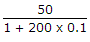.

7.

In figure v1 = 8 V and v2 = 4 V. Which diode will conduct?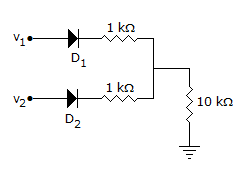A. D2 only B. D1 only C. Both D1 and D2 D. Neither D1 nor D2

Explanation:

D1 will conduct and the output voltage will be about 7 V. Therefore D2 will be reverse biased and will not conduct.

8.

The load impedance ZL of a CE amplifier has R and L in series. The phase difference between output and input will be

 A. 180° B. 0 C. more than 90° but less than 180° D. more than 180° but less than 270°

Explanation:

It is 180° for purely resistive load and between 180° and 270° for R-L load.

9.

Gain of the amplifier is 'A'. Then the I/P impedance and O/P impedance of the closed loop amplifier shown below would be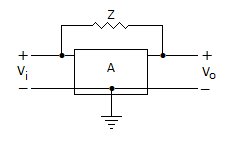A.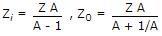B.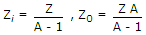C.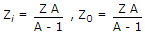D.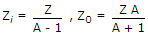Explanation:

By using Miller theorem circuit can be redrawn as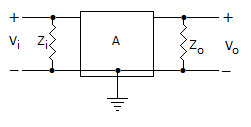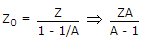.

10.

If an amplifier with gain of - 1000 and feedback factor β = - 0.1 had a gain change of 20% due to temperature, the change in gain of the feedback amplifier would be

 A. 10% B. 5% C. 0.2% D. 0.01%

Explanation: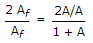As we know, Gain with feedback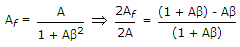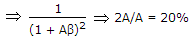A = - 1000, β = -0.1 .